# GSEB Solutions Class 11 Physics Chapter 5 Laws of Motion

Gujarat Board GSEB Textbook Solutions Class 11 Physics Chapter 5 Laws of Motion Textbook Questions and Answers.

## Gujarat Board Textbook Solutions Class 11 Physics Chapter 5 Laws of Motion

### GSEB Class 11 Physics Laws of Motion Text Book Questions and Answers

Question 1.
Give the magnitude and direction of the net force acting on:

1.  a drop of rain falling down with a constant speed.
2.  a cork of mass 10 g floating on water.
3. a kite skillfully held stationary in the sky.
4. a car moving with a constant velocity of 30 km/h on a rough road.
5. a high-speed electron in space far from all gravitating (material) objects, and free of electric and magnetic fields.

1. Since the drop of rain is falling downward with a constant speed, so the acceleration of the rain drop is zero. Hence according to Newton’s first law of motion, net force on the drop is zero i.e. a = 0, ∴ from F = ma, F = 0.

2. As the cork is floating in water, so the weight of the cork is balanced by upthrust (equal to weight of water displaced). Therefore net force on the cork is zero.

3. Since the kite is held stationary, so its acceleration is ~ zero. Hence according to Newton’s first law of motion, the net force acting on the kite is zero.

4. Since the car is moving with a constant velocity, so its acceleration is zero. Hence according to Newton’s first law of motion, the net force acting on the car is zero.

5. As there are no electric and magnetic fields and no material (gravitating) objects around, so no force (gravitational/electric/magnetic) is acting on the electron, so net force on it is zero.Question 2.
A pebble of mass 0.05 kg is thrown vertically upwards. Give the direction and magnitude of the net force on the pebble:
(a) during its upward motion
(b) during its downward motion
(c) at the highest point where it is momentarily at rest.
Do your answers change if the pebble was thrown at an angle of 45° with the horizontal direction? Ignore air resistance?
We know that whenever an object is thrown vertically upwards or it moves vertically downwards, the gravitational pull of Earth gives uniform acceleration a = + g = 9.8 ms-2 in the vertically downward direction.
If m = mass of the object, then net force on it = mg
Here m = 0.05 kg = mass of pebble.

(a) ∴ force on the pebble = mg (∵ a = g)
= 0.05 × 9.8
= 0.49N (Acts vertically downwards)

(b) Net force on the pebble = mg (∵ a = g)
= 0.05 × 9.8
= 0.49N
(Acts vertically downwards)

(c) When the stone is at the highest point then also the net force (= mg) acts in vertically downward direction
∴ Net force on the pebble = mg
= 0.05 × 0.8
= 0.49N
= 0.50N
If the pebble was thrown at an angle of 45° with the horizontal direction, then it will have horizontal and vertical components of velocity which don’t affect the force on the pebble. Hence our answers will not change in any case. However in case (c), the pebble will not be at rest at the highest point. It will have a horizontal component of velocity at this point.Question 3.
Give the magnitude and direction of the net force acting on a stone of mass 0.1 kg?
(a) just after it is dropped from the window of a stationary train,
(b) just after it is dropped from the window of a train running at a constant velocity of 36 km/h,
(c) just after it is dropped from the window of a train accelerating with 1 ms-2,
(d) lying on the floor of a train which is accelerating with 1 ms-2, the stone being at rest relative to the train. Neglect air resistance throughout.
Mass of stone, m = 0.1 kg
(a) When the stone is dropped from the window of a stationary train, it falls freely with an acceleration a = +g.
∴ Net force on the stone = mg
= 0.1 × 9.8
= 0.98N
= 1N and it acts vertically downwards.

(b) When the train is running at a constant velocity of 36 kmh-1,
then acceleration of the train = 0 in horizontal direction, so no force acts on the stone due to the this motion. When the stone is dropped from the window of this train, then it is again falling freely, so
net force on the stone = its own weight
= mg = 0.98N = 1N
and it acts vertically downwards.

(c) When the train is accelerating with 1ms-2, then following two forces act on the stone:
(i) Due to initial acceleration of 1 ms-2 of the train and it acts in the horizontal direction.
(ii) Due to its acceleration of 9.8ms-2 when dropped from the train which acts vertically downward.
∴ If F1 and F2 be the forces acting on the stone along horizontal and vertical directions:
Then F1 = ma
= 0.1 × 1
= 0. IN and
F2 = mg
= 0.1 × 9.8
= 0.98N
If F be the net force acting on the stone, then
F = $$\sqrt{F_{1}^{2}+F_{2}^{2}}$$
= $$\sqrt{(0.1)^{2}+(0.98)^{2}}$$
= 0.985N = 0.1N

Direction of F: Let θ be the angle made by F with F1
∴ tan θ = $$\frac{\mathrm{F}_{2}}{\mathrm{~F}_{1}}$$ = $$\frac{0.98}{0.1}$$ = 9.8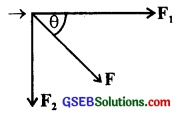= tan 84° 12′
∴ θ = 84° 12′ or 5° 48′ with vertical
i.e. F acts 5° 48′ with the vertical direction.

Aliter:
Here, when once the stone is dropped from the train, force F1 is to do nothing with the motion of the stone i.e. F1 becomes zero, so the net force on the stone = F2 = 0.98N = IN.
It acts vertically downward.

(d) F = ma = 0.1 × 1
=0.1N
and it gets along the direction of train.Question 4.
One end of a string of length l is connected to a particle of mass m and the other to a small peg on smooth horizontal table. If the particle moves in a circle with speed v, the net force on the particle (directed towards the centre) is:

1. T
2. T – $$\frac{{mv}^{2}}{l}$$
3. T + $$\frac{{mv}^{2}}{l}$$
4. 0

1. The net force on the particle is T, because tension T provides the necessary centripetal force i. e.T = $$\frac{{mv}^{2}}{l}$$

Question 5.
A constant retarding force of 50N is applied to a body of mass 20kg moving initially with a speed of 15ms-1. How long does the body take to stop?
We are given that
Force acting on the body, F = – 50 N
(-ve sign shows that it is retarding force)
m = mass of the body = 20 kg
u = initial velocity of body = 15ms1
v = Final velocity of the body
= 0
t = ?
Using relation,
F = ma
we get a = $$\frac{F}{m}$$
or a = – $$\frac{50}{20}$$
= – 2.5ms-2 (retardation)
using relation, v = u + at
we get 0 = 15 + (-2.5)t
or 2.5t = 15
∴ t = $$\frac{15}{2.5}$$ = 6s = t = 6s.

Question 6.
A constnat force acting on a body of mass 3.0 kg changes its speed from 2.0 ms-1 to 3.5 ms-1 in 25s. The direction of motion of the body remains unchanged. What is the magnitude and direction of the force?
m = 3 kg
u = 2 ms-1
v = 3.5 ms-1
t = 25 s
F = constant force acting on the body = ?
Let a = acceleration produced in the body
Using the relation,
v = u + at,
we get a = $$\frac{v-u}{t}$$ = $$\frac{3.5-2}{25}$$
= $$\frac{1.5}{25}$$ = $$\frac{15}{250}$$ms-2.
∴ Using the relation,
F = ma
we get the magnitude of the force as:
F = 3 × $$\frac{15}{250}$$ = 0.18N
The direction of the force is along the direction of motion.Question 7.
A body of mass 5 kg is acted upon by two. perpendicular forces 8N and 6N. Give the magnitude and direction of the acceleration of the body?
m = mass of the body = 5 kg
F1 = one force acting on the body = 8N
F2 = force ⊥to F1, acting on the body = 6N
θ = angle between F1 and F2 = 90°
a = acceleration of the body 0 = ?
To find a let us first find net force on the body.
Let F be the net force acting on the body.Magnitude of F:
According to parallelogram law of vector addition, the magnitude of F is given by= 10N

Direction of F:
Let a be the angle made by F with F1
∴ From rt ∠d triangle OAC,
tan α = $$\frac{AC}{OA}$$ = $$\frac{{F}_{2}}{{~F}_{1}}$$ = $$\frac{6}{8}$$ = 0.7500
= tan 36°53′
∴ α = 36°53′
= 37° with the direction of 8N
This is the direction of resultant force and hence the direction of acceleration of the body
Now a = $$\frac{F}{m}$$ = $$\frac{10}{5}$$ = 2 ms-2
∴ magnitude of acceleration = 2 ms-2 and acts in the direction of resultant force.

Question 8.
The driver of a three wheeler moving with a speed of 36 km/h sees a child standing in the middle of the road and brings his vehicle to rest in 4.0s just in time to save the child. What is the average retarding force on the vehicle? The mass of the three wheeler is 400 kg and the mass of the driver is 65 kg?
Initial speed of the three wheeler,
u = 36 km-1
36 × $$\frac{5}{18}$$ ms-1
= 10 ms-1
Final speed of the three wheeler,
v = 0
Time, t = 4s
F = average retarding force = ?
Total mass m = mass of three wheeler + mass of driver
= 400 + 65
= 465 kg
Using, the relation, v = u + at
we get a = $$\frac{v-u}{t}$$ = $$\frac{0-10}{4}$$
= $$\frac{-5}{2}$$ ms2 = -2.5 ms-2
– ve sign shows that it is retardation
F = m (-a)
= 465 × $$\frac{5}{2}$$ = 1162.5N 2
= 1.16 × 103N
= 1.2 × 103N.Question 9.
A rocket with a lift – off mass 20,000 kg is blasted upwards with an initial acceleration of 5.0 ms-2N. Calculate the initial thrust (force) of the blast?
m = mass of rocket = 20,000 kg
= 2 × 104 kg
a = acceleration of rocket
= 5 ms-2 in upward direction
g = 9.8 ms-2
Let T be the intial thrust acting upward = ?
W = mg = weight of rocket acting downward
∴ equation of motion becomes,
T – mg = ma
or T = mg + ma
= m(g + a) = 2 × 104(9.8 + 5)
= 14.8 × 2 × 104
= 29.6 × 104N
T = 2.96 × 105N = 3.0 × 105N

Aliter:
m = 2 × 104 kg= 2 × 104 × 5 + 2 × 104 × 9.8
= 105 + 1.96 × 105
= 2.96 × 105N
= 3 × 105N.

Question 10.
A body of mass 0.40 kg moving initially with a constant speed of 10 ms-1 to the north is subject to a constant force of 8.0 N directed towards the south for 30 s. Take the instant the force is applied to be t = 0, the position of the particle at that time to be x = 0 and predict its position at t = -5s, 25s, 100s?
Initial speed of the particle, u = 10 ms-1
Direction of motion = Northm = mass of particle 0.40 kg
F = constant force applied on the particle towards south = -8N
– ve sign shows that it is retarding force
mass of the particle, m = 0.4 kg
time for which force is applied, t = 30 s
Let us find

(i) position of the particle at t = – 5 s
from t = 0 to t = – 5 s, the particle moves towards north
if x1 = distance covered by the particle, then
x1 = ut
= 10 × (-5) = -50 m
(∵ u = 10 ms-1, t = -5s)

(ii) position of the particle at t = 25s
Let x25 = distance covered by the particle in 25s
If a = acceleration produced in the particle towards south for 0 ≤ t ≤ 30s
a = $$\frac{F}{m}$$ = $$\frac{-8}{0.40}$$ = $$\frac{80}{4}$$
or a = -20ms-2 for 0 ≤ t ≤ 30s
Using relation, s = ut + $$\frac{1}{2}$$at2 ……………………… (i), we get
x25 = 10 × 25 + $$\frac{1}{2}$$(-20) × (25)2
= 250 – 6250
= -6000 m
or x25 = – 6 km
As F acts for 30 seconds,
so if x30 be the distance covered by the particle in 30s, then from (i), we get
x300 = 10 × 30 + $$\frac{1}{2}$$(-20)(30)2
= 300 – 9000
= – 8700 m
If v = velocity of the particle after 30s, then using the equation
v = u + at ………………. (ii)
v = 10 + (-20) × 30
= 10 – 600
= – 590 ms-1
The particle will now move with a constant speed of -590 ms-1 as the force acts for 30s

(iii) Position at t = 100s
Then x100 be the distance covered after 100s,
Then x100 = x30 + x70 ………………….. (iii)
where x30 = – 8700 m ……………….. (iv)
ans x70 is the distance covered by the particle with – 590 ms-1 speed in time = 70s
∴ x70 = (-590) × 70 (∵ x = ut) …………………… (v)
∴ from (iii), (iv) and (v), we get
x100 = – 8700 – 41300
= – 50000 m
= – 50 km
So the distance travelled by the particle in -5s, 25 and 100s are -50 m, – 5 k, – 50 km respectively.Question 11.
A truck starts from rest and accelerates uniformly with 2ms-2. At t = 10s, a stone is dropped by a person standing on the top of the truck (6m high from the ground). What are the (a) velocity and (b) acceleration of the stone at t = 11s? (Neglect air resistance)?
Initial velocity, u = 0, a = 2.0 ms-2
t = 10 s
Let v be the velocity of truck when the stone is dropped from it after t = 10s.
∴ using the relation,
v = u + at, we get
v = 0 + 2.0 × 10 = 20 ms-1

(a) Horizontal velocity of the stone when it is dropped from the truck is vx = v = 20 ms-1. As air resistance is neglected, so vx = constant.

Motion in the vertical direction:
Initial velocity of the stone, vy = 0 at t = 10s.
acce., ay = g = 9.8 ms-2
time t = 11 – 10 = 1s
If vy be velocity of the stone after 1 sec of drop (i. e. at t = 11 s),
then Vy = uy + ayt
= 0 + 9.8 × 1
= 9.8 ms-2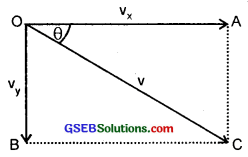If v be the velocity of the stone after 11 s is given by
v = $$\sqrt{v_{x}^{2}+y_{y}^{2}}$$
= $$\sqrt{(20)^{2}+(9.8)^{2}}$$
= 22.4 ms-1
Let θ be the angle which the resultant velocity OC of the stone makes with the horizontal direction OA i.e. with vx, then from ∆OAC,
= tan 26° 12′
θ = 26.2°

(b) At the moment, the stone is dropped from the truck the horizontal force on the stone is zero, so
ax = 0
and ay = acce. along vertical direction
= + g = 9.8 ms-2
which acts in downward direction.
∴ If a = resultant acceleration of the stone, then
a = $$\sqrt{a_{x}^{2}+a_{y}^{2}}$$
= $$\sqrt{0+(9.8)^{2}}$$
or a = 9.8 ms-2
and it acts vertically downward.Question 12.
A bob of mass 0.1 kg hung from the ceiling of a room by a string 2m long is set into oscillation. The speed of the bob at its mean position is 1 ms-1. What is the trajectory of the bob if the string is cut when the bob is:
(a) at one of its extreme positions.
(b) at its mean position.
(a) We know that at each extreme position, the instantaneous velocity of the bob is zero. If the string is cut at the external position, it is under the action of ‘g’ only, hence the bob will fall vertically downwards.
(b) When the bob is at the mean position, it is affected by gravity. At mean position the bob is having a velocity of 1ms-1 along the tangent to the arc which is in the horizontal direction. If the string is cut at the mean position, the bob will behave as a horizontal projectile. Hence it will follow a parabolic path.

Question 13.
A man of mass 70 kg stands on a weighing scale in a lift which is moving:
(a) upwards with uniform speed of 10 ms-1.
(b) downwards with a uniform acceleration of 5 ms-2.
(c) upwards with uniform acceleration of 5 ms-2.
What would be the readings on the scale in each case?
(d) What would be the reading if the lift mechanism failed and it hurtled down freely under gravity?
(a) m = 70 kg, g = 9.8 ms-2
The weighing machine in each case measures the reaction R i.e. the apparent weight.
When the speed of the lift is uniform it becomes inertial frame of reference for the man i.e. its acceleration a = 0.
So the weighing machine will show his weight i.e.
R = W = mg = 70 × 9.8
= 686 N
We acts vertically downward and R acts vertically upward
∴ Reading on the scale = 686 N = 70 kg

(b) When the lift moves downward with an acceleration
a = 5 ms-2
∴ Resultant force acts vertically downward
i.e. F = mg – R
or ma = mg – R
or R = mg – ma = m(g – a)
= 70(9.8 – 5)
= 70 × 4.8 = 336N
∴ reading of the scale = 336N = $$\frac{336}{9.8}$$kg = 34.29 kg

(c) In this case the lift is moving upward with an acceleration,
a = 5 ms-2
∴ Resultant force acting upward is
F = R – mg
or R = ma + mg
= m(g + a)
= 70(9.8 + 5)
= 14.8 × 70
= 1036N
∴ Reading of the scale = 1036 N = $$\frac{1036}{9.8}$$ = 105.7 kg

(d) In this case the lift mechanism failed and it falls freely under gravity i.e. with an acceleration
a = g = 9.8 ms-2
∴ R = m(g – a) = m(g – g) = 0
Thus the reading on the scale is zero
This is the state of weightlessness.Question 14.
Figure below shows the position-time graph of a particle of mass 4 kg. What is the
(a) force on the particle for t < 0, t > 4s, 0 < t < 4s?
(b) impulse at t = 0 and t = 4s? (Consider one dimensional motion only).mass of particle = m = 4g
(a)
(i) For t < 0, the position time graph is 0’0 which means displacement of the particle is zero i.e. the particle is at rest at the origin. So acceleration and hence force on the particle must be zero.

(ii) For t > 4s, the position-time graph AA’ is parallel to time axis. Therefore, the particle remains at a distance of 3m from the origin i.e. it is at rest. Hence no force is acting on the particle for this interval i.e. F = 0.

(iii) For 0 < t < 4s, the particle is changinig its position continuously. The position-time graph (OA) during this interval represents uniform motion of the particle i.e. it moves with a constant speed and thus its acceleration is zero. Hence
F = ma = 0 during this interval.

(b) (i) Impulse at t = 0
We know that
impulse = change in momentum
= mv – mu
= m(v – u) …………………. (i)
Here u = 0 as trie particle is at rest before t = 0 .
After t = 0, the particle has a constant velocity v where
v = slope of line OA = velocity at point O and point A

(ii) Impulse at t = 4s
Before t = 4s, the particle has a constant
velocity u = $$\frac{3}{4}$$ms-1
After t = 4s, the particle is at rest i.e. v = 0
∴ By definition of impulse, we get
Impulse = mv – mu
= m(v – u)
= 4(0 – 0.75) [By usingequn.(3)]
= – 3 kg ms-1.

Question 15.
Two bodies of masses 10 kg and 20 kg respectively kept on a smooth, horizontal surface are tied to the ends of a light string. A horizontal force F = 600N is applied to (i) A, (ii) B along the direction of string. What is the tension in the string in each case?
F = 600N
Let m1 = 10 kg
and m2 = 20 kg
be the masses lying on a frictionless horizontal table.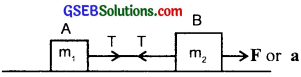Let T be the tension in the string and ‘a’ be the acceleration of the system, in the direction of force applied.

(a) When force is applied on the heavier mass.
Then equations of motion of A and B are:
m1a = T …………………….. (i)
m2a = F – T ………………….. (ii)
Dividing (ii) by (i), we get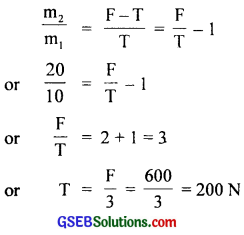(b) When the force is applied on lighter mass:Let T be the tension in the string in this case
Then equations of motion of A and B areQuestion 16.
Two masses 8 kg and 12 kg are connected at the two ends of a light inextensible string that goes over a frictionless pulley. Find the acceleration of the masses and the tension in the string when the masses are released?
Let m1 and m2 be the masses suspended at the ends of a light inextensible string passing over the pulley.
∴ m1 = 8 kg,
m2 = 12 kg
Let T = tension in the string = ?
a = common acceleration with which m1 moves upward and m2 moves downward = ?
The eqns. of motion of m1 and m2 are given by
T – m1g = m1a ……………………. (i)
and m2g – T = m2a ……………………… (ii)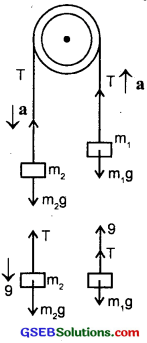Adding (i) and (ii), we get
(m2 – m1)g = (m1 + m2)a
∴ a = $$\frac{\left(m_{2}-m_{1}\right) g}{m_{1}+m_{2}}$$ ………………….. (iii)
∴ from (i) and (iii), we get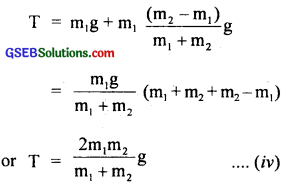Putting m1 = 8 kg and m2 = 12kg and g = 10 ms-2, in equations (iii), (iv) we get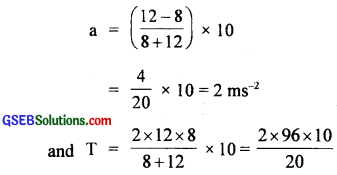= 96 N.
∴ a = 2 ms-2, T = 96N.

Question 17.
A nucleus is at rest in the laboratory frame of reference. Show that if it disintegrates into two smaller nuclei the products must be emitted in opposite directions?
Let m = mass of the nucleus at rest.
u = its initial velocity = 0 as it is at rest.
Also let m1, m2 be the masses of the two smaller nuclei also called product nuclei and v1, v2 be their respective velocities.
If Pi and pr be the initial and final momentum of the nucleus and the two nuclei respectively, then
Pi = mu = 0 …………………… (1)
and pr = m1v1 + m2v2 ………………… (2)
Now according to the law of conservation of linear momentum, we know that
Pi = Pr
or 0 = m1v1 + m2v2
or m2v2 = -m1v1
or v2 = –$$\frac{\mathrm{m}_{1} \mathbf{v}_{1}}{\mathrm{~m}_{2}}$$ ……………….. (3)
The negative sign in equation (3) shows that v1 and v2 are in opposite directions i.e. the two smaller nuclei are emitted in opposite directions.Question 18.
Two billiard balls each of mass 0.05 kg moving in opposite directions with speed 6 ms-1 collide and rebound with the same speed. What is the impulse imparted to each ball due to the other?
Mass of each ball,
m = 0.05 kg
Speed of each ball,
v = 6 ms-1
∴ Initial momentum of each ball
pi = mv
= (0.05) (6)
= 0.30 kg ms-1
As after collision, the direction of velocity of each ball is reversed on rebounding
∴ Final momentum of each ball = m (-v)
pf = 0.05 (-6)
= – 0.30 kg ms-1
∴ Impulse imparted to each ball = pf – pi
= change in momentum of each ball
= – 0.30 – (0.30)
= – 0.60 kg ms-1
or magnitude of impulse imparted by one ball due to collision with the other = 0.6 kg ms-1. The two impulses are opposite in direction.

Question 19.
A shell of mass 0.020 kg is fired by a gun of mass 100 kg. If the muzzle speed of the shell is 80 ms-1, what is the recoil speed of the gun?
Here, mass of gun, M = 100 kg
mass of shell, m = 0.02 kg
speed of snell, v = 80 ms-1
recoil of gun, V = ?
As initially both the gun and the shell are at rest before firing, so the initial momentum (pi) of the system = 0
Final momentum (pf) of the system after firing ……………………. (i)
= MV + mv
= 100V + 0.02 × 80 …………………. (ii)
∴ According to the law of conservation of linear momentum,
Pi = Pr
or 0 = 100v + 1.6
or 100v = – $$\frac{1.6}{100}$$
= 0.016 ms-1
∴ recoil speed of gun = V = 0.016 ms-1
= 1.6 cms-1Question 20.
A batsman deflects a ball by an angle of 45° without changing its initial speed which is equal to 54 kmh-1. What is the impulse imparted to the ball? (mass of the ball is 0.15 kg).
Let the ball of mass m moves along AO with an initial speed u and hits the bat ML and is deflected along OB with ∠AOB = 45°.
Let ON be the normal to the bat i. e. the deflecting surface such that
∠AON = ∠NOB = θ = $$\frac{45°}{2}$$ = 22.5°
The initial and final velocities of the ball can be resolved into rectangular components. It is clear from the figure here that the components of speed and hence the momentum along ML remain constant while along the normal they act opposite to each other and hence change in momentum takes place.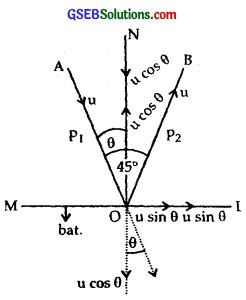∴ Component of initial velocity along NO = u cos θ
Component of final velocity along ON = – u cos θ
where negative sign shows that final velocity acts opposite to initial speed i.e. velocity along the vertical is just reversed.
∴ initial momentum of the ball along NO = mu cos θ
and final momentum of the ball along ON = – mu cos θ
Thus impulse imparted to the ball = change in the linear momentum of the ball
= mu cos θ – (- mu cos θ)
= 2 mu cos θ ……………….. (1)
here m = 0.15 kg, u = 54 kmh-1
= 54 × $$\frac{5}{18}$$ = 15ms-1 ……………………… (2)
θ = 22.5°
∴ From (1) and (2), we get
Impulse = 2 × 0.15 × 15 × cos 22.5°
= 4.5 × 0.9239
= 4.16 kg ms-1
= 4.2 kg ms-1 .Question 21.
A stone of mass 0.25 kg tied to the end of a string is whirled round in a circle of radius 1.5 m with speed 40 rev./min in a horizontal plane. What is the tension in the string? What is the maximum speed with which the stone can be whirled around if the string can withstand a maximum tension of 200N?
Frequency of revolution of stone,
v = 40 rev./min = $$\frac{40}{60}$$ r/s
mass of stone, m = 0.25 kg
radius of circle, r = 1.5 m
angular speed of the stone, ω = 2πv
= 2π × $$\frac{40}{60}$$ = $$\frac{4π}{3}$$ rad s-1
T = tension in the string = ?
Tmax = maximum tension in the string
= 200 N
vmax = maximum speed of the stone = ?
The centipetal force is provided by the tension (T) in the string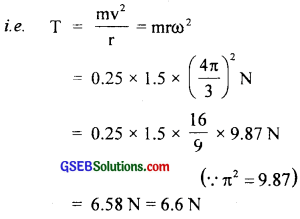As the string can withstand a maximum tension of 200 N= 34.64 ms-1 = 35.0 ms-1
∴ T = 6.6 N, vmax = 35.0 ms-1.

Question 22.
If in 5.21, the speed of the stone is increased beyond the maximum permissible value, and the string breaks suddenly, which of the following correctly describes the trajectory of the stone after the string breaks:
(a) the stone moves radially outwards,
(b) the stone flies off tangentially from the instant the string breaks,
(c) the stone flies off at an angle with the tangent whose magnitude depends on the speed of the particle?
(b) Correctly describes the trajectory of the stone after the string breaks i.e. the stone flies off tangentially from the instant the string breaks.
The velocity always acts tangentially to the circle at each point in the circular motion. At the time, the string breaks, the particle continues to move in the tangential direction according to Newton’s first law of motion.Question 23.
Explain why:
(a) a horse cannot pull a cart and run in empty space,
(b) passengers are thrown forward from their seats when a speeding bus stops suddenly,
(c) it is easier to pull a lawn mower than to push it.
(d) a cricketer moves his hands backwards while holding a catch.
(a) While trying to pull a cart, a horse pushes the ground backwards with a certain force at an angle. The ground offers an equal reaction in opposite direction; on the feet of the horse. The forward component of this reaction is responsible for the motion of cart. In empty space, there is no reaction and hence horse cannot pull the cart and run.

(b) This is due to inertia of motion possessed by the passengers in a speeding bus.

(c) Consider the free body diagram in the two cases, the lawn mower is represented by the point O.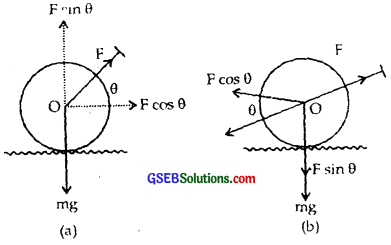The forces acting on the lawn mower are (i) applied force F, (ii) weight of the mower W downward (iii) force of friction acting opposite to the direction of motion (iv) reaction of ground on the mover (R).
In case of pushing the mower, the horizontal component of push F cos θ in forward direction and vertical component F sin θ in downward direction. The total downward force = W + F sin θ hence the reaction
R = W + F sin θ (Newton’s third law of motion) and force of friction,
f = µR = µ(W + F sin 0) (∵ W = mg)
where µ = coefficient of friction coefficient
If P be the net forward force, then
P = F cos θ – f
= F cos θ – µ(mg + F sinθ) ………………….. (i)
In case of pull, the forward component of pull = F cos θ
and vertical component = F sin θ
If R’ be the normal reaction in this case,
then R’ = W – F sin θ
= mg – F sin θ
∴ Is f’ be the force of friction in this case, then net force P is given by
P’ = F cos θ – f’
= F cos θ – µR’
= F cos θ – µ(mg – F sin θ) ……………………….. (ii)
From (i) and (ii), we see that P’ > P; Hence it is easier to pull than to push the lawn mover.

(d) While holding a catch, the impulse received by the hands, F × t = change in momentum of the ball is constant. By moving his hands backwards, the cricketer increases the time of impact (t) to complete the catch. As t increases, F decreases and as a reaction, the ball hurts his hands lesser.